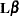ESTIMATE Statement

ESTIMATE <'label'> estimate-specification <(divisor=n)>
<, ...<'label'> estimate-specification <(divisor=n)> >
< / options>
;

The ESTIMATE statement provides a mechanism for obtaining custom hypothesis tests. Estimates are formed as linear estimable functions of the form. You can perform hypothesis tests for the estimable functions, construct confidence limits, and obtain specific nonlinear transformations.

Table 90.4 summarizes important options in the ESTIMATE statement.

Table 90.4 Important ESTIMATE Statement Options

Option

Description

Construction and Computation of Estimable Functions

Specifies a list of values to divide the coefficients

Suppresses the automatic fill-in of coefficients for higher-order effects

Tunes the estimability checking difference

Degrees of Freedom and p-values

Determines the method for multiple comparison adjustment of estimates

ALPHA=Determines the confidence level ()

Performs one-sided, lower-tailed inference

Adjusts multiplicity-corrected p-values further in a step-down fashion

Specifies values under the null hypothesis for tests

Performs one-sided, upper-tailed inference

Statistical Output

Constructs confidence limits

Displays the correlation matrix of estimates

Displays the covariance matrix of estimates

Prints thematrix

Produces a jointor chi-square test for the estimable functions

Specifies the seed for computations that depend on random numbers

For details about the syntax of the ESTIMATE statement, see the section ESTIMATE Statement of Chapter 19, Shared Concepts and Topics.